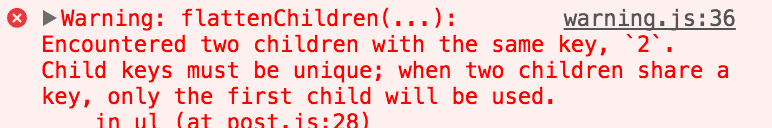# React中使用collections时key的重要性详解

React 不会 render 重复的 keys

``````
const nums = [1, 2, 3, 5, 2]; // 它有两个元素的值是相等的``````

``````
<ul>
{nums.map(num => <li key={num}>{num}</li>)}
</ul>``````

``````
<ul>
<li key="1">1</li>
<li key="2">2</li>
<li key="3">3</li>
<li key="5">5</li>
<li key="2">2</li>
</ul>``````

``````
<ul>
<li>1</li>
<li>2</li>
<li>3</li>
<li>5</li>
</ul>``````

React 给出了以下warningReact 会 re-render 某个 key 指向的内容发生变化的元素

``````
const users = [
];

users.map((u, i) =>

render 的结果如下

``````
<div key="bob">bob</div>
<div key="sue">sue</div>``````

``````
const users = [
];``````

render 的结果将会改变如下

``````
<div key="joe">joe</div>
<div key="bob">bob</div>
<div key="sue">sue</div>``````

``````
users.map((u, i) =>

• 将 id 为 "1" 的元素从 "bob" 更改为 "joe"
• 将 id 为 "2" 的元素从 "sue" 更改为 "bob"
• 新增一个 id 为 "3" 的元素, 他的值为 "sue"